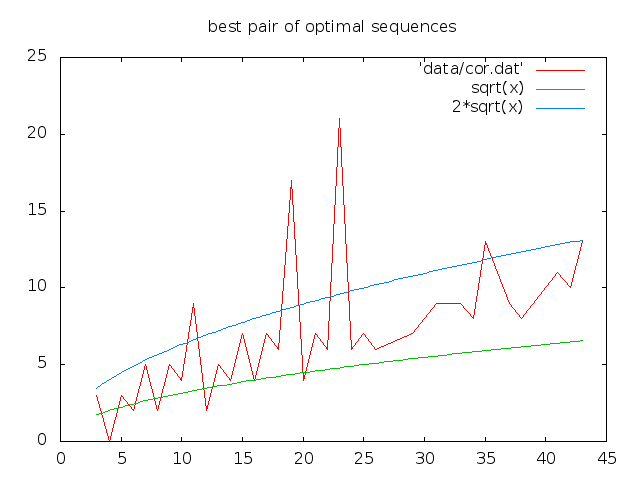# Best Pair of Binary Sequences

This page reports on the best pair of binary sequences for the auto and cross correlation point of views.
Philippe Langevin, Last modification February 28th 2014.

### Correlation

Let n be a positive integer, f end g two binary sequences of length n i.e. two mapping from the cyclic group Z/nZ into {-1,+1}. The cross correlation between f and g at t is defined by

fxg (t) = sum_{ y - x = t } f(x) g(y)
in other words, the scalar product between the vector f and the t-shift of g. One defines the correlation values :
R(f) = max { f x f (t) | 0 <> t in Z/LZ } and I(f,g) = max { f x g (t) | t in Z/LZ }

### Terminology

To a given sequence f we associate a polynomial of degree less than n in a natural way

f(T) = sum_{k} f(k) T^k

We have two products :
f(T) f( 1/T ) = sum_{k} A_k(f) T^k and f(T) f( 1/T ) = sum_{k} C_k(f) T^k modulo (T^n - 1)

The coefficients C_k(f) are nothing but the autocorrelation values of f, the A_k(f) are called aperiodic correlation values. A sequence with A_k(f) smaller than one in absolute (for k>0) is called a Barker sequence. Such sequences could be useful for radar applications but one conjectures the non existence of Barker sequences of length greater than 13. A sequence with C_k(f) equals to zero for all k>0 is called perfect, one conjectures the non existence of such combinatorial object for n>4. It is easy to see that
C_k(f) = n modulo 4

a reason why one says a binary sequence has optimal correlation when
sup_{k>0} | fxf( k)| = 4, 3, 2, 1 according to the congruence of n modulo 4 is : 0, 1, 2, 3.

### Objective

Provides datas about the best pair of binary sequences i.e. pair (f,g) of optimal sequences minimizing the correlation parameters :

theta( n ) := min sup { f x g (t) | t in ZnZ}
where both f and g are optimal sequences.

### Methode

One can see the correlation values of a sequence do not depend on shift and change of sign. For the small values of n ( less than 40 ), one can use a naive approach to compute the distribution of optimal sequences, here are the codes we used :

[src/countps.c] [src/genopt.c] [src/bpbs.c] [cpu.txt]
The running time for n=41 was about 14 hours, using 18 cores of the above architecture below, the same when offloading on xeon phi.
```[langevin@mejean COUNTPS]\$ cat job.585.out
[Offload] [MIC 0] [File]            countps.c
[Offload] [MIC 0] [Line]            99
[Offload] [MIC 0] [Tag]             Tag 0
[Offload] [HOST]  [Tag 0] [CPU Time]        51451.540086(seconds)
[Offload] [MIC 0] [Tag 0] [CPU->MIC Data]   28 (bytes)
[Offload] [MIC 0] [Tag 0] [MIC Time]        51210.619694(seconds)
[Offload] [MIC 0] [Tag 0] [MIC->CPU Data]   28 (bytes)

L=41 (1) alpha=3 opt=4842
[langevin@mejean COUNTPS]\$ cat job.586.out

L=41 (1) alpha=3 opt=4842
[langevin@mejean COUNTPS]\$ cat job.586.err
time=14:12:57
```

### Results

The distribution of optimal sequences up to shift, up to change of sign with length less or equal to 43. The best pairs of optimal sequences are in the opt-file and bp-file. The correlation picture :### References

 The Cross-Correlation of Binary Sequences With Optimal Autocorrelation, Cunsheng Ding and Xiaohu Tang, ieee transactions on information theory, vol. 56, no. 4, april 2010.